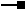# ITopologicalOperator5 Interface

Provides additional information on non-simple geometries.

## Members

Name DescriptionBoundary The boundary of this geometry. A polygon's boundary is a polyline. A polyline's boundary is a multipoint. A point or multipoint's boundary is an empty point or multipoint.Buffer Constructs a polygon that is the locus of points at a distance less than or equal to a specified distance from this geometry.Clip Constructs the intersection of this geometry and the specified envelope.ClipDense Constructs the intersection of this geometry and the specified envelope; densifies lines in output contributed by the clipping envelope.ClipEx Constructs the intersection of this geometry and the specified envelope.ClipToDomain Clips the geometry to the domain of the spatial reference. Useful for ensuring that buffers can be fit within the spatial domain of the feature class to which they are being added.ConstructBuffers Constructs a set of buffers at various distances. More efficient than calling Buffer repeatedly on the same geometry. This method is intended for internal use only.ConstructUnion Defines this geometry to be the union of the inputs. More efficient for unioning multiple geometries than calling Union repeatedly.ConvexHull Constructs the convex hull of this geometry.Cut Splits this geometry into a part left of the cutting polyline, and a part right of it.Cut2 Divides a geometry into multiple partsDifference Constructs the geometry containing points from this geometry but not the other geometry.GeoNormalizeEx Shifts longitudes, if need be, into a continuous range of 360 degrees.Intersect Constructs the geometry that is the set-theoretic intersection of the input geometries. Use different resultDimension values to generate results of different dimensions.IntersectMultidimension Constructs the set-theoretic intersection of the inputs. The results are returned in a geometry bag with one element per result dimension.IsKnownSimple Indicates whether this geometry is known (or assumed) to be topologically correct.IsKnownSimple Indicates whether this geometry is known (or assumed) to be topologically correct.IsSimple Indicates whether this geometry is known (or assumed) to be topologically correct, after explicitly determining this if the geometry is not already known (or assumed) to be simple.IsSimpleEx Determines why a geometry is not simple. Currently only implemented for polygons.QueryClipped Redefines clippedGeometry to be the intersection of this geometry and the clipping envelope.QueryClippedDense Redefines clippedGeometry to be the intersection of this geometry and the clipping envelope; densifies lines in the output contributed by the clipping envelope.Simplify Makes this geometry topologically correct.SymmetricDifference Constructs the geometry that contains points from either but not both input geometries.TopologyCache Provides a handle to the TopologyCache.Union Constructs the geometry that is the set-theoretic union of the input geometries.

### ITopologicalOperator5.ClipEx Method

Constructs the intersection of this geometry and the specified envelope.

``````Public Sub ClipEx ( _
ByVal clipperEnvelope As IEnvelope, _
ByVal bIncludeBoundary As Boolean _
)
``````
``````public void ClipEx (
IEnvelope clipperEnvelope,
bool bIncludeBoundary
);
``````

#### Description

TheClipEx method clips the geometry of the feature that is receiving the method call to the input clipperEnvelope, with the added option of whether you want to include segments lying on the boundary.

#### Remarks

ITopologicalOperator5 methods must be applied on high-level geometries only. High-Level geometries are point, multipoint, polyline and polygon. To use this interface with low-level geometries such as segments (Line, Circular Arc, Elliptic Arc, Bezier Curve), paths or rings, they must be wrapped into high-level geometry types.

## Inherited Interfaces

Interfaces Description
ITopologicalOperator4 Provides additional information on non-simple geometries.
ITopologicalOperator3 Provides additional information on non-simple geometries.